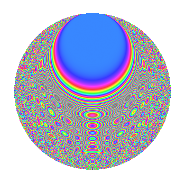# Properties

 Label 1575.2.bfLevel 1575 Weight 2 Character orbit bf Rep. character $$\chi_{1575}(551,\cdot)$$ Character field $$\Q(\zeta_{6})$$ Dimension 292 Sturm bound 480

# Related objects

## Defining parameters

 Level: $$N$$ = $$1575 = 3^{2} \cdot 5^{2} \cdot 7$$ Weight: $$k$$ = $$2$$ Character orbit: $$[\chi]$$ = 1575.bf (of order $$6$$ and degree $$2$$) Character conductor: $$\operatorname{cond}(\chi)$$ = $$63$$ Character field: $$\Q(\zeta_{6})$$ Sturm bound: $$480$$

## Dimensions

The following table gives the dimensions of various subspaces of $$M_{2}(1575, [\chi])$$.

Total New Old
Modular forms 504 316 188
Cusp forms 456 292 164
Eisenstein series 48 24 24

## Trace form

 $$292q + 3q^{2} + 3q^{3} + 139q^{4} + 6q^{6} - 3q^{9} + O(q^{10})$$ $$292q + 3q^{2} + 3q^{3} + 139q^{4} + 6q^{6} - 3q^{9} + 3q^{12} + 3q^{13} - 3q^{14} - 123q^{16} + 9q^{17} - q^{18} + 6q^{19} - 18q^{21} - 2q^{22} + 12q^{24} + 18q^{26} - 9q^{27} + 10q^{28} + 24q^{29} - 21q^{31} - 39q^{32} + 30q^{33} - 18q^{34} - 60q^{36} + 3q^{37} + 66q^{38} - 18q^{39} + 6q^{41} + 21q^{42} + 6q^{43} + 15q^{44} - 4q^{46} + 21q^{47} - 45q^{48} + 14q^{49} - 18q^{51} - 24q^{53} - 42q^{54} - 18q^{56} + 21q^{57} - 2q^{58} - 12q^{59} - 6q^{61} - 24q^{62} - 20q^{63} - 180q^{64} + 45q^{66} - 8q^{67} + 108q^{68} + 48q^{69} + 49q^{72} + 6q^{73} + 48q^{76} + 6q^{77} + 9q^{78} - 14q^{79} + 21q^{81} - 30q^{83} - 69q^{84} + 63q^{87} - 22q^{88} - 27q^{89} + 5q^{91} - 42q^{92} + 48q^{93} + 3q^{94} - 126q^{96} + 3q^{97} + 33q^{98} - 6q^{99} + O(q^{100})$$

## Decomposition of $$S_{2}^{\mathrm{new}}(1575, [\chi])$$ into newform subspaces

The newforms in this space have not yet been added to the LMFDB.

## Decomposition of $$S_{2}^{\mathrm{old}}(1575, [\chi])$$ into lower level spaces

$$S_{2}^{\mathrm{old}}(1575, [\chi]) \cong$$ $$S_{2}^{\mathrm{new}}(63, [\chi])$$$$^{\oplus 3}$$$$\oplus$$$$S_{2}^{\mathrm{new}}(315, [\chi])$$$$^{\oplus 2}$$

## Hecke Characteristic Polynomials

There are no characteristic polynomials of Hecke operators in the database